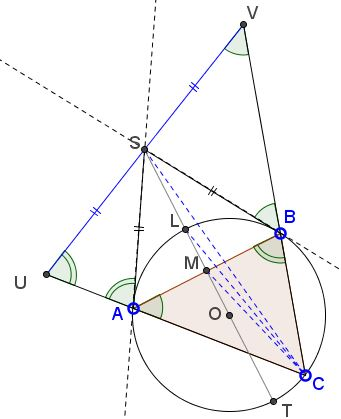# Symmedian and the TangentsWhat is this about? A Mathematical Droodle

ExplanationA symmedian through one of the vertices of a triangle passes through the point of intersection of the tangents to the circumcircle at the other two vertices.The proof is based on a known fact: the locus of the midpoints of the antiparallels to a side of a triangle is the summedian through the opposite vertex. Draw an antiparallel through S - the point of intersection of the two tangents to the circumcircle of the triangle ABC at A and B. Let it meet the extended sides AC and BC at U and V, respectively.Then the triangles USA and VSB are isosceles, so that

SU = SA and
SV = SB.

SA = SB,

as two tangents from a point to a circle. We conclude that S is the midpoint of UV. Therefore, S belongs to the locus of all such midpoints. Since the locus is the symmedian - a straight line - through the vertex C, CS is bound to be that symmedian.

### References

1. R. Honsberger, Episodes in Nineteenth and Twentieth Century Euclidean Geometry, MAA, 1995.### Symmedian